# Convert an Array to an Object using JavaScriptLast updated: Jan 7, 2023
5 min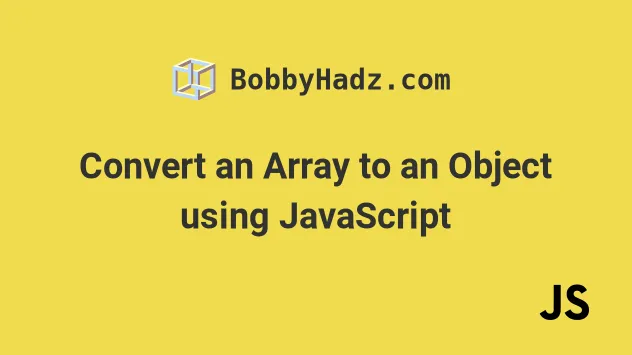## #Convert an Array to an Object using JavaScript

The most common ways to convert an array to an object in JavaScript are:

1. `Object.assign()` method - the keys are the array indexes and the values are the array elements.
2. `Array.forEach()` method - enables you to specify custom keys.
3. `Object.fromEntries()` method - transforms an array of key-value pairs into an object.
Which approach you should pick depends on whether you need to use the array's indexes as object keys or you need to use custom keys.

## #Convert an Array to an Object using Object.assign()

Use the `Object.assign()` method to convert an array to an object.

The `Object.assign` method takes a target and source objects as parameters, applies the properties from the sources to the target and returns the result.

index.js
```Copied!```const arr = ['zero', 'one', 'two'];

const obj1 = Object.assign({}, arr);
console.log(obj1); // 👉️ {0: 'zero', 1: 'one', 2: 'two'}
``````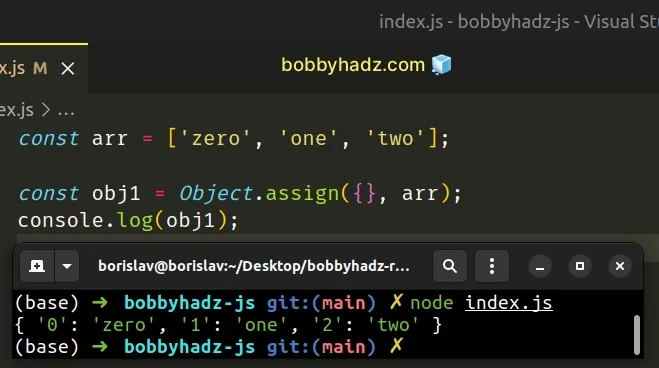We passed the following 2 arguments to the `Object.assign` method:

1. target object - the object to which we apply the sources' properties
2. source object(s) - objects containing the properties we want to apply
The method returns an object where the keys are the array indexes and the values are the array elements.

## #Convert an Array to an Object using Array.forEach()

This is a three-step process:

1. Declare a variable and initialize it to an empty object.
2. Use the `Array.forEach()` method to iterate over the array.
3. On each iteration, add the element as a key-value pair to the object.
index.js
```Copied!```const arr = ['zero', 'one', 'two'];

const obj = {};

arr.forEach((element, index) => {
obj[`key\${index}`] = element;
});

// 👇️️ {'key0': 'zero', 'key1': 'one', 'key2': 'two'}
console.log(obj);
``````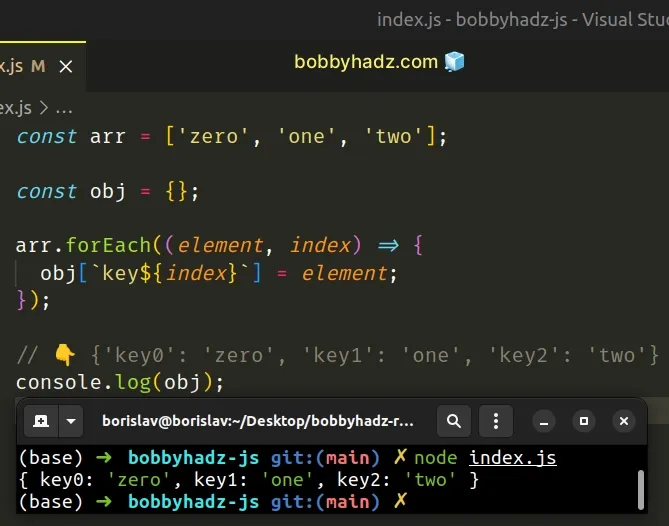The function we passed to the Array.forEach method gets called with each element in the array.

The method also gives us access to the index of the current iteration.

This approach is a bit more manual, however, it allows you to name the keys of the new object.

You aren't able to name the keys of the object if you use the `Object.assign()` approach from the previous subheading.

The function we passed to the `Array.forEach()` method gets called with each element and the index, so you could also use the `index` as a key.

index.js
```Copied!```const arr = ['zero', 'one', 'two'];

const obj = {};

arr.forEach((element, index) => {
obj[index] = element;
});

// 👇️️ { '0': 'zero', '1': 'one', '2': 'two' }
console.log(obj);
``````

## #Convert an Array to an Object using Spread syntax (...)

Alternatively, you can use the spread syntax (...).

The spread syntax will unpack the values of the array into a new object, where:

1. The indexes of the array are the object's keys.
2. The elements in the array are the object's values.
index.js
```Copied!```const arr = ['zero', 'one', 'two'];

const obj = {...arr};
console.log(obj); // 👉️ {0: 'zero', 1: 'one', 2: 'two'}
``````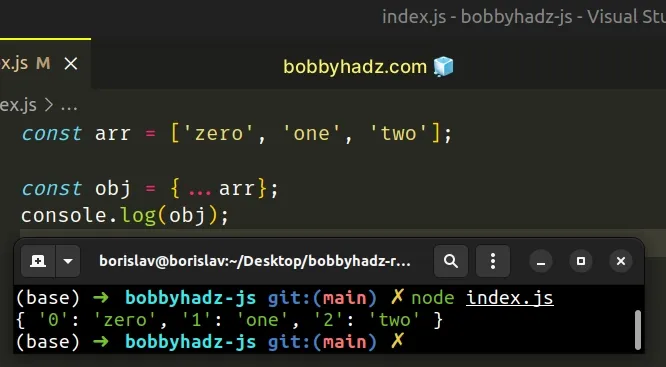We used the spread syntax (...) to unpack the array into an object.

The spread (...) syntax can be used with any iterable, such as an array, string, `Set`, etc.

Similarly to the first approach, the indexes of the array become the object's keys and the elements become the object's values.

## #Convert an Array to Object using Object.fromEntries()

To convert an array of key-value pairs to an object:

1. Pass the array to the `Object.fromEntries()` method.
2. The `Object.fromEntries()` method transforms an array of key-value pairs into an object and returns the result.
index.js
```Copied!```const arr = [
['age', 30],
];

const obj = Object.fromEntries(arr);

console.log(obj); // 👉️ { name: 'bobby hadz', age: 30 }
``````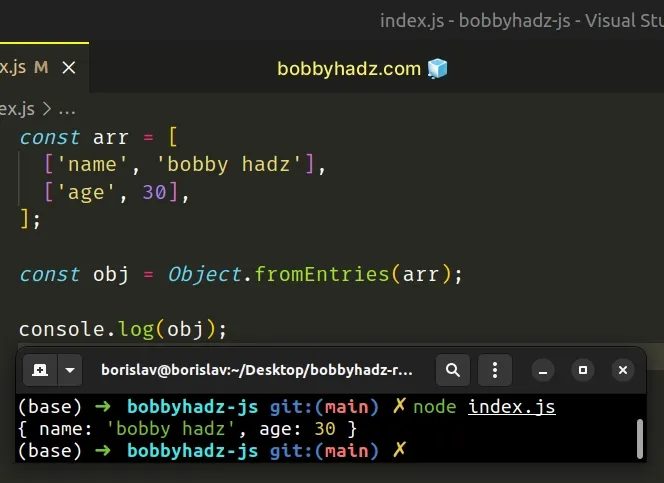Notice that we have a two-dimensional array this time.

The nested arrays contain 2 elements each - a key and a value.

The Object.fromEntries transforms a list of key-value pairs into an object.

This only works if you have a two-dimensional array where each subarray contains 2 elements - the key and the value.

## #Convert an Array to an Object using Array.reduce()

This is a two-step process:

1. Use the `Array.reduce()` method to iterate over the array.
2. On each iteration, assign a new key-value pair to the accumulated object.
index.js
```Copied!```const arr = ['zero', 'one', 'two'];

const obj = arr.reduce((accumulator, value, index) => {
return {...accumulator, ['key' + index]: value};
}, {});

// 👇️️ {'key0': 'zero', 'key1': 'one', 'key2': 'two'}
console.log(obj);
``````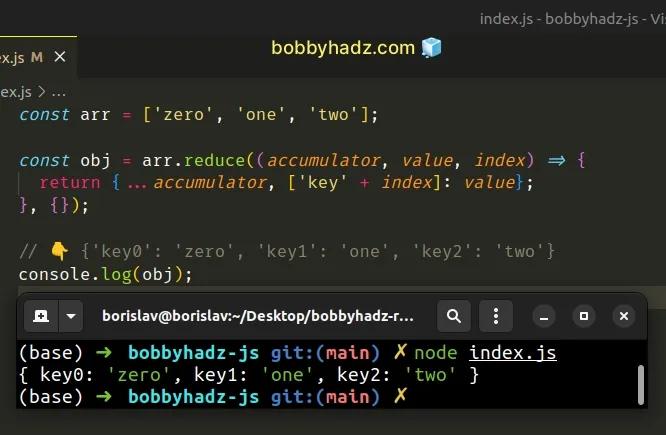The function we passed to the Array.reduce method gets called with each element in the array.

We set an empty object as the initial value for the `accumulator` variable.

On each iteration, we assign a new key-value pair to the object and return the value of the `accumulator` variable.

This approach also allows you to assign custom key names to the object.

We used the string `"key"` + the index, however, you can adjust this as needed.

## #Convert an Array of Objects to an Object using `Object.fromEntries()`

To convert an array of objects to an object:

1. Use the `Array.map()` method to iterate over the array.
2. Return an array of a key and a value on each iteration.
3. Use the `Object.fromEntries` method to convert the array to an object.
index.js
```Copied!```const arr = [
{name: 'country', value: 'Austria'},
{name: 'age', value: 30},
{name: 'city', value: 'Vienna'},
];

const obj = Object.fromEntries(
arr.map(obj => [obj.name, obj.value])
);

// 👇️ { country: 'Austria', age: 30, city: 'Vienna' }
console.log(obj);
``````

The function we passed to the Array.map() method gets called with each element in the array.

On each iteration, we return an array containing a key and a value.

index.js
```Copied!```const arr = [
{name: 'country', value: 'Austria'},
{name: 'age', value: 30},
{name: 'city', value: 'Vienna'},
];

// 👇️ [ [ 'country', 'Austria' ], [ 'age', 30 ], [ 'city', 'Vienna' ] ]
console.log(arr.map(obj => [obj.name, obj.value]));
``````

The `map()` method returns a new array containing the values returned from the callback function.

The last step is to pass the array of key-value pairs to the `Object.fromEntries()` method to transform it into an object.

## #Convert an array to an Object using a `for` loop

This is a three-step process:

1. Declare a new variable that stores an empty object.
2. Use a `for` loop to iterate over the array.
3. On each iteration, add a key-value pair to the object.
index.js
```Copied!```const obj = {};

const arr = ['bobby', 'hadz', 'com'];

for (let index = 0; index < arr.length; index++) {
obj[index] = arr[index];
}

// 👇️ { '0': 'bobby', '1': 'hadz', '2': 'com' }
console.log(obj);
``````

We used a basic `for` loop to iterate over the array.

On each iteration, we add the current key-value pair to the object using the index as the key.

When using this approach, you can also specify custom keys.

## #Conclusion

When converting an array to an object, use the:

• `Object.fromEntries()` method if you need to convert an array of key-value pairs to an object.
• `Object.assign()` method or the spread syntax (...) if the array's indexes should be the object's keys.
• `Array.forEach()` or `Array.reduce()` method if you need to customize the keys.
• `Array.map()` and `Object.fromEntries()` methods if you need to convert an array of objects to an object.